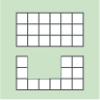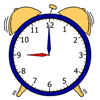#### You may also like### Next Size Up

The challenge for you is to make a string of six (or more!) graded cubes.### Area and Perimeter

What can you say about these shapes? This problem challenges you to create shapes with different areas and perimeters.### Order, Order!

Can you place these quantities in order from smallest to largest?

# Discuss and Choose

##### Age 5 to 11Challenge Level

Some good suggestions came to us from pupils from many different countries. The answers I used were from a Nature Statistics web site. Mya from Ricards Lodge High School, UK sent in the following ideas for number $7$. She showed a good use of animal knowledge and of mathematical skills.

I think that I might have a solution for number $7$.
The question is ... Adult elephants need to drink about $100$/$200$/$400$ litres of water a day. I think that it is ... $100$l.
An average adult human should drink about $2$ litres of water a day (coffee and tea don't count). An average ADULT human weights about between $65$/$80$ kg. So the median of the two numbers is $78$, so it should be approximately $1$l per $39$ kg ($39$ is half $78$), for a human.
So bearing that in mind if an average adult elephant however weights $4.6$ tons, so converting that into kg that would be $4600$kg.($1$ ton is equal to $1000$kg.)  So that is more than $70$ times more than an average human weight ... because $65x70$ would give you $4550$kg - only $50$kg short of $4600$kg.
So if you multiply that by $70$ then multiply the amount of water (litres) by $70$ ... so you would get $140$.  Rounding it to the nearest hundred you get $100$l !!
I think that is right.

Ella, from the same school, sent in these answers:

$1) 500$ million
$2) 4.5$kg
$3) 13-14$ hours
$4) 400$ million
$5) 10-14$ years
$6) 20$ individuals
$7) 100$ litres
$8) 170$cm
$9) 5$ times as thick
$10) 180$kg
$11) 8$km
$12) 20$ hours

1. Domestic cats sleep on average 8-9/13-14/18-19 hours a day.
The children thought that cats sleep 13-14 hours a day because adult humans tend to sleep 8 - 9 hours and children sleep about 12 hours each night. The children who have their own cats noticed that their cats also sleep during the daytime, not just at night, so they think they sleep longer than humans. They didn't think they slept 18-19 hours a day as this would mean they would only be awake for 5 or 6 hours a day.

2. Adult elephants need to drink about 100/200/400 litres of water a day.
The children thought that 400 was the "best" number here. We talked about the fact that children probably drink 1-1.5 litres of water each day, and that adults probably drink about 2 litres. They thought that perhaps the reason elephants were so slow when they moved was because their tummies were so full up of water. They also knew that an elephant must drink a lot of water because they are so large.

3. On average, cats weigh 1.5/4.5/8kg.
Some children in the class have cats and can lift them up. We used classroom weights and asked one girl to pick them up. She could easily carry 1.5kg, but when we added 3 more kilograms, she said it was hard to hold them all. She said it weighed more than her cat, and she wouldn't be able to lift her cat if it weighed that much. So the children decided that cats must weigh 1.5kg.

Aadil from Riverside Primary School, UK sent in these good suggestions as answers, many of which were correct:

$1$. $500$ million
$2$. $4.5$kg
$3$. $18-19$ hours a day
$4$. $600$ million
$5$. $10-14$ years
$6$. $30$ individuals
$7$. $200$ litres
$8$. $170$ cm
$9$. $5$x
$10$. $250$kg
$11$. $16$km

Betina, Luiza and Katrina from the British School in Barra, Brazil sent in these solutions, written here in the same order as their names:

$1$ $500, 500, 500$
$2$ $4.5, 4.5, 4.5$
$3$ $13-14, 13-14, 13-14$
$4$ $600, 400, 400$
$5$ $18-20, 10-14, 10-14$
$6$ $12, 12, 12$
$7$ $400, 200, 200$
$8$ $220, 170, 170$
$9$ $5, 10, 10$
$10$ $330, 250, 250$
$11$ $8, 8, 8$
$12$ $15, 20, 20$

We also had answers from Germain, Mount Zion School, U.S.A., Julia from TromsÃ˜
School in Norway and Harry and Fin from Jebel Ali School in U.A.E.

The answers that I used to set the challenge were:

$1) 500$

$2) 4$ to $5$

$3) 13$ to $14$

$4) 400$

$5) 10$ to $14$

$6) 12$

$7) 200$

$8) 170$

$9) 10$x

$10) 180$

$11) 8$

$12) 20$